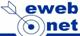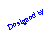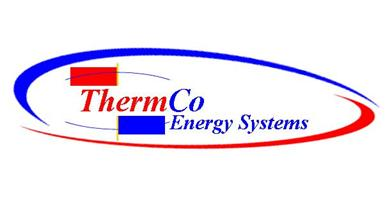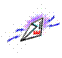Retro Fitting is thinking   GREEN – RECYCLING          REDUCING CARBON FOOTPRINTWhat’s EER or SEER? Heating and Air Conditioning Ratings
 The new rating, S.E.E.R. (Seasonal Energy Efficiency Ratio), is an alternative to the original E.E.R., and to better approximate the actual cooling cost of operation of an "appliance" based on the installed climate zone. S.E.E.R. is a different efficiency rating than E.E.R. and is based on residential air conditioner usage patterns. S.E.E.R. includes a rating at a different temperature than E.E.R. and also includes performances such as conditions of cycling (off/on) thus includes cycling losses.   Currently all HVAC unit specification sheets are required to show only S.E.E.R. ratings. E.E.R. and S.E.E.R. are two ways to determine the cost of operation of an HVAC unit. A 10 S.E.E.R. became the first federally mandated minimum efficiency in 1992 for residential split systems and 9.7 S.E.E.R. for single package systems in 1993. This new federal efficiency standard preempts any state standards.   To sum it up: S.E.E.R. is a seasonal rating most typifying average residential usage in the US. E.E.R. is at a single rating point and more represents peak load rating. High rated S.E.E.R. Air cooled HVAC unit rating falls as the temperature gets hotter and in turn cost more to operate.   S.E.E.R. conventional air-cooled units are rated at 83 degrees. The operating efficiency is lowered by two points for each ten-degree rise in the temperature above the 83-degree test standard. A 12 SEER air-cooled unit will operate at an 8 to 9 SEER on a 105-degree day. A water cooled system will operate in the 17 to 19 EER range, providing an operational savings in excess of 60%! The hotter it gets the better a water-cooled unit works!   Other considerations to factor in is the Urban Heat Island effect.     One size DOES NOT fit all!
 Study by: Arizona State University  Funded by:   ASHRAE
 Home Depot Report             Sacramento CaliforniaIn 1975 the Air Conditioning & Refrigeration Institute (ARI) introduced the E.E.R. (Energy Efficiency Ratio) for the purpose of rating the cooling efficiency of HVAC units. E.E.R. equaled the rated cooling output of an HVAC unit in BTU's per hour divided by the rated input of energy in watts of electricity, at specific humidity, and temperature input/output conditions.   To calculate EER you just divide Total Capacity by the Total KW energy usage.   The definition of Energy Efficiency Ratio is an efficiency measurement of BTUH cooling output versus power (watts) input or BTUH/WATT at a specific set of indoor and outdoor dry bulb and wet bulb temperature conditions.   The ARI rating point has been at 80 db./67 wb. indoor and 95 db./75 wb. outdoor temperatures. No seasonality was taken into consideration. The climate zones across the U.S. vary, as do seasonal conditions from one zone to another. This means the seasonal conditions affect the value and must be weighed when interpreting an HVAC unit's E.E.R. rating.   As explained in our performance data, Florida and Arizona have different summer conditions, which affect the performance and resulting in the cooling energy savings for the user of any HVAC unit.   1978 the US Congress passed a law requiring labeling of certain "appliances" (HVAC units under 65,000 btuh cooling) with an efficiency rating that took into consideration how certain variables (seasonality) affect cooling BTUH output, watts input, and an average cost of operation for the cooling side of a residential HVAC unit. S.E.E.R. (Seasonal Energy Efficiency Ratio)   S.E.E.R. is an efficiency rating, somewhat like how miles per gallon (MPG) is used to rate automobile efficiency. For the HVAC, S.E.E.R. follows the same rationale. E.E.R. is more like the highway rating of MPG on a car and S.E.E.R. is more like the city driving MPG rating of a car.
 Here are two simple water cooled projects.
 Thermco Energy Systems